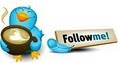#Calculated Member - Measure1*Measure2/Sum(Measure1)• ### Question

•Hi Experts,

I've two measures - Amount and Factor against some dimensions A,B and C.

When I drag and drop the above measures with respect to Dimension A as shown below...

Dimension-A          Amount           Factor

xxx-123                 100                   2

XXX-565                 120                  4

Total                       320                 6

I want one calculated member which is Amount*Factor/SUM(Amount). For example as shown below...

Dimension-A          Amount           Factor    CalculatedMember

xxx-123                 100                   2           100*2/320

XXX-565                 120                  4            120*4/320

Total                       320                 6           100*2/320 + 120*4/320

--NoorBi

Wednesday, November 13, 2013 7:02 AM

•It is difficult to answer properly without some more details, but I will give it a shot.

Suppose there is a fact table having DimA, DimB, DimC, Amount and Factor.

﻿Now, if I want the result to be at the level of the fact table, best option is to make a calculated member in the fact table with the below formula

`=[Amount] * [Factor]`and then you can use a calculated field (measure) with the formula

=sum(Fact[Option 1])

Now if you just want the formula to apply at the values of Dimension A, then you can make a calculated field (measure) with the formula below

`Option 2:=sumx(values(DimA[Dim A]), sum(Fact[Amount]) * sum(Fact[Factor]))`Cheers,
Jason | www.SqlJason.com